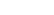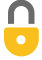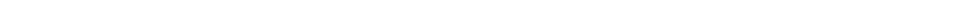Problem 22
Q

# If the speed of a car is increased by 50%, by what factor will its minimum braking distance be increased, assuming all else is the same? Ignore the driver’s reaction time.A
$d_2 = 2.25d_1$In order to watch this solution you need to have a subscription.VIDEO TRANSCRIPT

This is Giancoli Answers with Mr. Dychko. Change in kinetic energy is net force times displacement and we can also say it's the final kinetic energy minus the initial kinetic energy. But the final kinetic energy of this car will be zero because it comes to a stop and so we will say that net force times displacement, that's the stopping distance, equals the negative one-half mass times initial velocity squared. And we'll solve for the stopping distance d by dividing both sides by F net. F net is the breaking force because there's only going to be one horizontal force in the car when the brakes are applied and so that horizontal braking force is the net force and that force will be the same in both cases, regardless of what the car's speed is, because we are told to assume all else is the same. So the mass of the car is the same and the net force is the same. And so we have these two cases here; the stopping distance in case one where you have v initial one; and then the stopping distance in case two where the formula is exactly the same except you have v initial two. And we are told that v initial two is 50 percent more than v initial one. So 50 percent more means times by one and a half. So, we'll rewrite d 2 by substituting one and a half v initial one in place of v initial two; and we'll get this line here and then we'll take d 2 divided by d 1 to find out by what factor the distance has changed. So, we have d 2 copied here and then dividing by d 1 is the same as multiplying by the reciprocal of d 1. So, I have flipped over d 1 and rewrote it here as a multiplication and that makes it a little easier to see that most things cancel; 2 F net, the negatives cancel, the m's cancel and v initial one squared cancels and there we have, d 2 over d 1 is one and a half squared. So d 2 increases by a factor of two and a quarter times d 1, when the speed is increased by 50 percent. So there's a significant significantly more increase in the stopping distance than there is in the speed. This is a 125 percent increase so there you go.

Giancoli Answers, including solutions and videos, is copyright © 2009-2023 Shaun Dychko, Vancouver, BC, Canada. Giancoli Answers is not affiliated with the textbook publisher. Book covers, titles, and author names appear for reference purposes only and are the property of their respective owners. Giancoli Answers is your best source for the 7th and 6th edition Giancoli physics solutions.